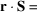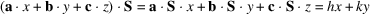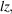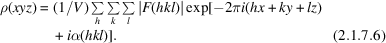International
Tables for
Crystallography
Volume F
Crystallography of biological macromolecules
Edited by E. Arnold, D. M. Himmel and M. G. Rossmann

International Tables for Crystallography (2012). Vol. F, ch. 2.1, p. 60   | 1 | 2 |

## Section 2.1.7. Calculation of electron density

J. Drentha*

aLaboratory of Biophysical Chemistry, University of Groningen, Nijenborgh 4, 9747 AG Groningen, The Netherlands
Correspondence e-mail: j.drenth@chem.rug.nl

### 2.1.7. Calculation of electron density

| top | pdf |

In equation (2.1.4.6),the wave(S) scattered by the crystal is given as the sum of the atomic contributions, as in equation (2.1.4.5)for the scattering by a unit cell. In the derivation of equation (2.1.4.5),it is assumed that the atoms are spherically symmetric (Section 2.1.4.3) and that density changes due to chemical bonding are neglected. A more exact expression for the wave scattered by a crystal, in the absence of anomalous scattering, isThe integration is over all electrons in the crystal.is the electron-density distribution in each unit cell. The operation on the electron-density distribution in equation (2.1.7.1)is called Fourier transformation, andis the Fourier transform of. It can be shown thatis obtained by an inverse Fourier transformation:In contrast tois not a continuous function but, because of the Laue conditions, it is only different from zero at the reciprocal-lattice points. In equation (2.1.4.6),is the product of the structure factor and three delta functions. The structure factor at the reciprocal-lattice points is F(h), and the product of the three delta functions is, the volume of one reciprocal unit cell. Therefore,in equation (2.1.7.2)can be replaced by, and equation (2.1.7.2)itself byIf x, y and z are fractional coordinates in the unit cell,+and an alternative expression for the electron density isInstead of expressing F(S) as a summation over the atoms [equation (2.1.4.5)],it can be expressed as an integration over the electron density in the unit cell:Becauseis a vector in the Argand diagram with an amplitudeand a phase angle,andBy applying equation (2.1.7.6),the electron-density distribution in the unit cell can be calculated, provided values ofandare known. From equation (2.1.6.1),it is clear thatcan be derived, on a relative scale, fromafter a correction for the background and absorption, and after application of the Lorentz and polarization factor:Contrary to the situation with crystals of small compounds, it is not easy to find the phase anglesfor crystals of macromolecules by direct methods, although these methods are in a state of development (see Part 16). Indirect methods to determine the protein phase angles are:

 (1) isomorphous replacement (see Part 12); (2) molecular replacement (see Part 13); (3) multiple-wavelength anomalous dispersion (MAD) (see Part 14).

From equation (2.1.7.5), it is clear that the reflectionsandhave the same value for their structure-factor amplitudes,, and for their intensities,, but have opposite values for their phase angles,, assuming that anomalous dispersion can be neglected. Consequently, equation (2.1.7.6)reduces toordenotes thatis excluded from the summation and that only the reflections, and not, are considered.

The two reflections,and, are called Friedel or Bijvoet pairs.

If anomalous dispersion cannot be neglected, the two members of a Friedel pair have different values for their structure-factor amplitudes, and their phase angles no longer have opposite values. This is caused by thecontribution to the anomalous scattering (Fig. 2.1.7.1).Macromolecular crystals show anomalous dispersion if the structure contains, besides the light atoms, one or more heavier atoms. These can be present in the native structure or are introduced in the isomorphous replacement technique or in MAD analysis.Figure 2.1.7.1 | top | pdf |An Argand diagram for the structure factors of the two members of a Friedel pair.representsand (−) represents.is the contribution to the structure factor by the non-anomalously scattering protein atoms andis that for the anomalously scattering atoms.consists of a real part with an imaginary part perpendicular to it. The real parts are mirror images with respect to the horizontal axis. The imaginary parts are rotated counterclockwise with respect to the real parts (Section 2.1.4.4). The result is that the total structure factors,and, have different amplitudes and phase angles. Reproduced with permission from Drenth (1999). Copyright (1999) Springer-Verlag.